# One-dimensional heat equation

Let a one-dimensional heat equation with homogenous Dirichlet boundary conditions and zero initial conditions be subject to spatially and temporally distributed forcing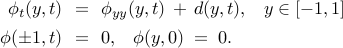The second derivative operator with Dirichlet boundary conditions is self-adjoint with a complete set of orthonormal eigenfunctions,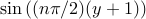,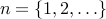. This information can be used to diagonalize operator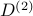which facilitates straightforward determination of the frequency response. For systems with spatially varying coefficients and non-normal generators the frequency response analysis is typically done numerically using finite dimensional approximations of the differential operators. For example, the pseudospectral method with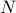collocation points can be used to transform the frequency response operator into an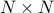matrix. However, for systems with differential operators of high order, spectral differentiation matrices may be poorly conditioned and implementation of boundary conditions may be challenging. In our method, numerical approximation of differential operators in the evolution equation is avoided by first recasting the system into corresponding two point boundary value problems and then using state-of-the-art techniques for solving the resulting boundary value problems with accuracy comparable to machine precision.

### Two point boundary value representations of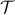,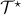, and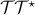Application of the temporal Fourier transform yields the two point boundary value representation of the frequency response operator,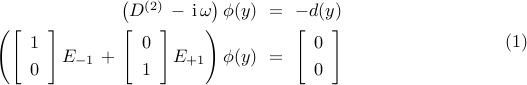where— imaginary unit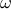— temporal frequency— second-order differential operator in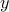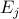— point evaluation functional at the boundary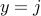.

We note that svdfr.m only requires the system's coefficients and boundary condition matrices appearing in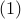to compute singular value decomposition of the frequency response operator. For completeness, we next show how to obtain the two point boundary value representations of the adjoint operatorand the operator. Furthermore, to illustrate the procedure used in our algorithm for computing singular value decomposition of the operator, we show how to convert a differential equation into an equivalent integral equation.

The two point boundary value representation for the adjoint of the frequency response operator,, is given by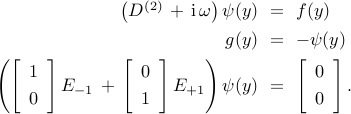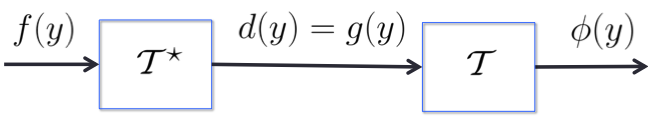Fig.: Block diagram of.

The representation of the operatoris obtained by combining the two point boundary value representations ofand. As illustrated in Fig., this can be achieved by setting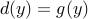in,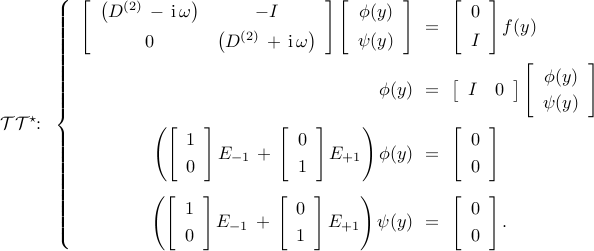Note that svdfr.m utilizes the integral form of a two point boundary value representation of. This procedure yields accurate results even for systems with high order differential operators and poorly-scaled coefficients.

### Integral form of a differential equation

We next illustrate the procedure for converting the differential equationinto its corresponding integral form. By introducing an auxiliary variable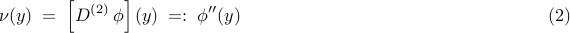and by integrating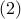we obtain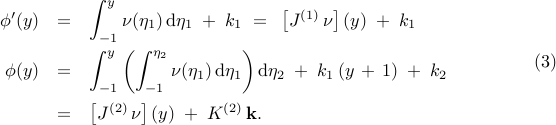Here,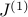and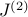denote the indefinite integration operators of degrees one and two, the vector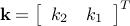contains the constants of integration which are to be determined from the boundary conditions, and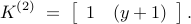The integral form of the 1D heat equation is obtained by substituting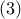into,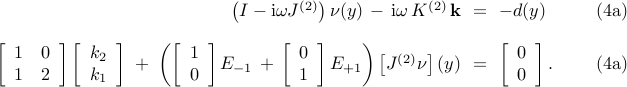Now, by observing that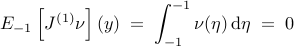we can use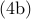to express the constants of integration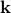in terms of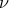,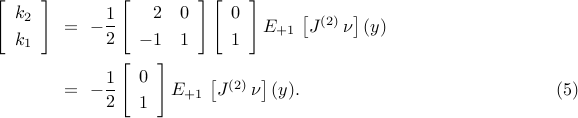Finally, substitution of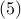into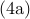yields an equation for,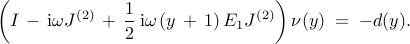This equation only contains indefinite integration operators and point evaluation functionals which are known to be well-conditioned.

### Matlab codes

```% The system parameters:
om = 0; % set temporal frequency to the value of interest

dom = domain(-1,1);     % domain of your function
y = chebfun('y',dom);
fone = chebfun(1,dom);      % one function
fzero = chebfun(0,dom);     % zero function

% The system operators can be constructed as follows:
A0{1} = [-1i*om*fone, fzero, fone];
B0{1} = -fone;
C0{1} = fone;

% The boundary condition matrices are given by:
Wa0{1} = [1, 0];   % 1*phi(-1) + 0*D^{(1)}*phi(-1) = 0
Wb0{1} = [1, 0];   % 1*phi(+1) + 0*D^{(1)}*phi(+1) = 0

% The singular values and the associated singular functions of the
% frequency response operator can be computed using the following code

Nsigs = 25;    % find the largest 25 singular values
LRfuns = 1;    % and associated left singular functions

[Sfun, Sval] = svdfr(A0,B0,C0,Wa0,Wb0,LRfuns,Nsigs);

% Analytical expressions for the singular values and corresponding singular
% functions are given by:
Sa = (4./(([1:Nsigs].*pi).^2)).';    % analytical singular values
Sf1 = sin((1/sqrt(Sa(1))).*(y+1));   % analytical solution of the first singular function
Sf2 = sin((1/sqrt(Sa(2))).*(y+1));   % analytical solution of the second singular function

% The absolute error of the first 25 singular values
norm(Sval - Sa)
```
```ans =

5.4288e-15

```

Singular values of the frequency response operator: svdfr versus analytical results.

```plot(1:Nsigs,Sval,'bx','LineWidth',1.25,'MarkerSize',10)
hold on
plot(1:Nsigs,Sa,'ro','LineWidth',1.25,'MarkerSize',10);
xlab = xlabel('n', 'interpreter', 'tex');
set(xlab, 'FontName', 'cmmi10', 'FontSize', 20);
h = get(gcf,'CurrentAxes');
set(h, 'FontName', 'cmr10', 'FontSize', 20, 'xscale', 'lin', 'yscale', 'lin');
```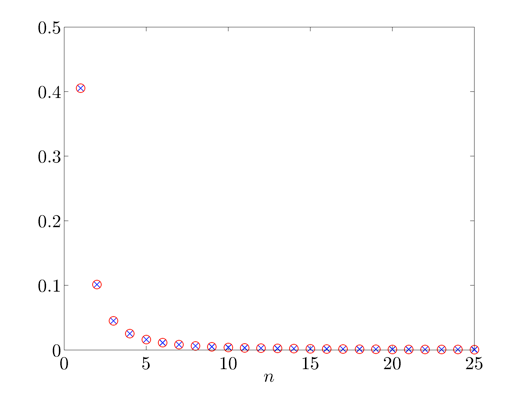Twenty five largest singular values of the frequency response operator.

The principal singular function: svdfr versus analytical results.

```hold off; plot(y,-Sfun(:,1),'bx-','LineWidth',1.25,'MarkerSize',10)
hold on; plot(y,Sf1,'ro-','LineWidth',1.25,'MarkerSize',10);
xlab = xlabel('y', 'interpreter', 'tex');
set(xlab, 'FontName', 'cmmi10', 'FontSize', 20);
h = get(gcf,'CurrentAxes');
set(h, 'FontName', 'cmr10', 'FontSize', 20, 'xscale', 'lin', 'yscale', 'lin');
axis tight
```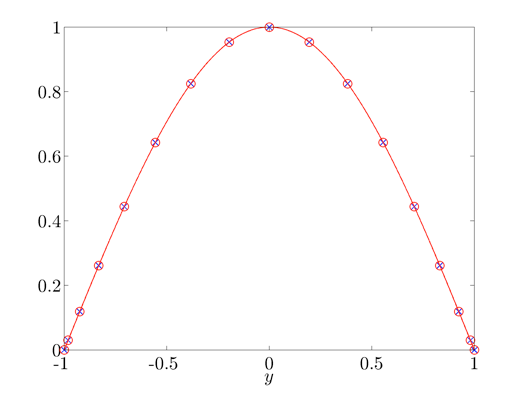The principal singular function of the frequency response operator.

The singular function corresponding to the second largest singular value: svdfr versus analytical results.

```hold off; plot(y,Sfun(:,2),'bx-','LineWidth',1.25,'MarkerSize',10)
hold on; plot(y,Sf2,'ro-','LineWidth',1.25,'MarkerSize',10);
xlab = xlabel('y', 'interpreter', 'tex');
set(xlab, 'FontName', 'cmmi10', 'FontSize', 20);
h = get(gcf,'CurrentAxes');
set(h, 'FontName', 'cmr10', 'FontSize', 20, 'xscale', 'lin', 'yscale', 'lin');
axis tight
```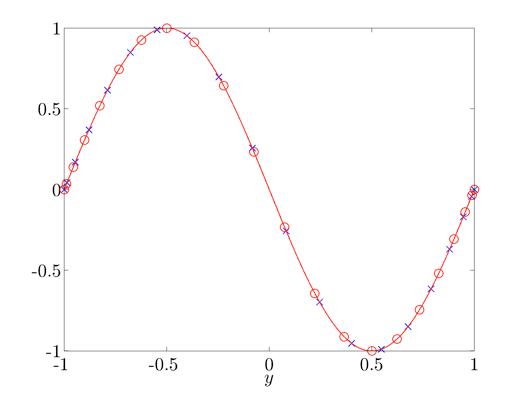The singular function of the frequency response operator corresponding to the second largest singular value.